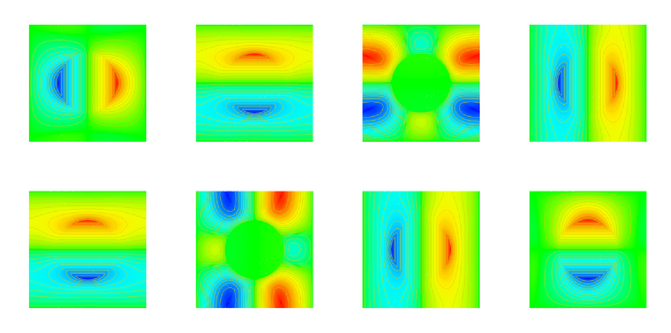Effective Elasticity tensor

# Calculation of an effective elasticity tensorAssume that we have a two-dimensional elastic medium with periodically distributed circular inlays consisting of a stiffer material. If these holes are small then the medium will behave on a macroscopic scale like a homogeneous medium without holes. The elasticity tensor of this medium can be calculated as follows:
• On the representative cell compute a tensor N of rank 3 which correspond to responses of the representative cell to different loads. Boundary conditions for this problem are periodic.
• Compute the effective tensor by evaluating the load functionals on the tensor N.
The picture above shows the 8 components of the tensor N for the 2D-case.

We used conforming finite elements of order 5 with nonlinear element mappings to approximate the tensor N. Solver for the linear problems was a W(1,1)-multigrid cycle with an overlapping block Gauss-Seidel smoother.

The history of this calculation was as follows (three of the 16 components are shown, due to symmetry properties the remaining ones can be obtained by a suitable permutation of indices):

Cells Unknowns Matrix entries A1111 A1212 A1221
9 1800 43060 4.1458940638 1.3176717343 1.2966840277
36 7200 176224 4.1412496929 1.3139564023 1.2979726371
144 28800 705600 4.1412384319 1.3139473004 1.2979716831
576 115200 2822400 4.1412383854 1.3139472825 1.2979716903
2304 460800 11289600 4.1412383853 1.3139472825 1.2979716903

Nicolas Neuss, 2017-01-31